21.2 (EAP/Beta)
21.1
20.2
20.1
19.2
19.1
18.2
18.1
17.2

# PivotSummaryType Enum

Lists values that specify the summary function types.

Namespace: DevExpress.Data.PivotGrid

Assembly: DevExpress.PivotGrid.v21.1.Core.dll

## Declaration

``````[ResourceFinder(typeof(ResFinder), "PropertyNamesRes")]
public enum PivotSummaryType``````

## Members

Name Description
Count

The number of values (excluding Null and DBNull values).

Sum

The sum of the values.Min

The smallest value.

Max

The largest value.

Average

The average of the values.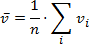StdDev

An estimate of the standard deviation of a population, where the sample is a subset of the entire population.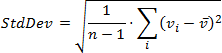StdDevp

The standard deviation of a population, where the population is all of the data to be summarized.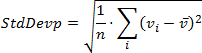Var

An estimate of the variance of a population, where the sample is a subset of the entire population.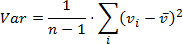Varp

The variance of a population, where the population is all of the data to be summarized.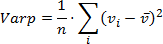Custom

Specifies whether calculations should be performed manually, by handling the CustomSummary event.

Not supported in OLAP and server mode.

CountDistinct

The number of distinct values. This function works only in the Optimized mode.

Median

The median of the values. A median is the number separating the higher half of a value range from the lower half. This function works only in the Optimized mode.

Mode

The mode of the values. A mode is the value that is repeated more often than any other. This function works only in the Optimized mode.

## Remarks

The PivotSummaryType type enumerates the values which represent the summary function types.